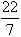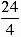Home MonkeyNotes Printable Notes Digital Library Study Guides Message Boards Study Smart Parents Tips College Planning Test Prep Fun Zone Help / FAQ How to Cite New Title Request

 4.7 Circles Perimeter of a circle is the circumference which is given by the formula. Perimeter of a circle = 2 p r where r is the radius, p =and has been discovered by ancient Greeks, by actually dividing the circumference of a circle by its diameter . Area of a circle = p r2 where ' r ' is the radius of the circle. Example 1 If the area of a square is 16 sqft. , find the length of each side . Solution: Area of a square = (length of one side)2 \ length of one side = 4 ft Example 2 If the perimeter of a square = 24 inches , What is its area ? Solution: If perimeter 24 inches, length of each side == 6 inches. \ Area = (6 inches)2 = 36 square inches. Example 3 The perimeter of a rectangle is 36 cm, find its area if the length of one side is 12 cm. Solution: Area = 72 cm2 Length of the rectangle = 12 and breadth = y \ 2 ´ 12 + 2 y = 36 24 + 2y = 36 or 2y = 12 \y = 6 cm Area = xy = 72 cm2 Example 4 The length of a rectangle is 10 cm and its perimeter is 30 cm. Find the area of this rectangle. Solution: Perimeter = 30 cm , length = 10 cm, breadth = y 2 ´ 10 + 2xy = 30 20 + 2y = 30 2y = 10 i.e. y = 5 \ area = 10 ´ 5 = 50 Index 4.1 Perimeter 4.2 Square 4.3 Rectangle 4.4 Parallelogram 4.5 Triangle 4.6 Trapezoids 4.7 Circles Chapter 5
 Search: All Products Books Popular Music Classical Music Video DVD Toys & Games Electronics Software Tools & Hardware Outdoor Living Kitchen & Housewares Camera & Photo Cell Phones Keywords: